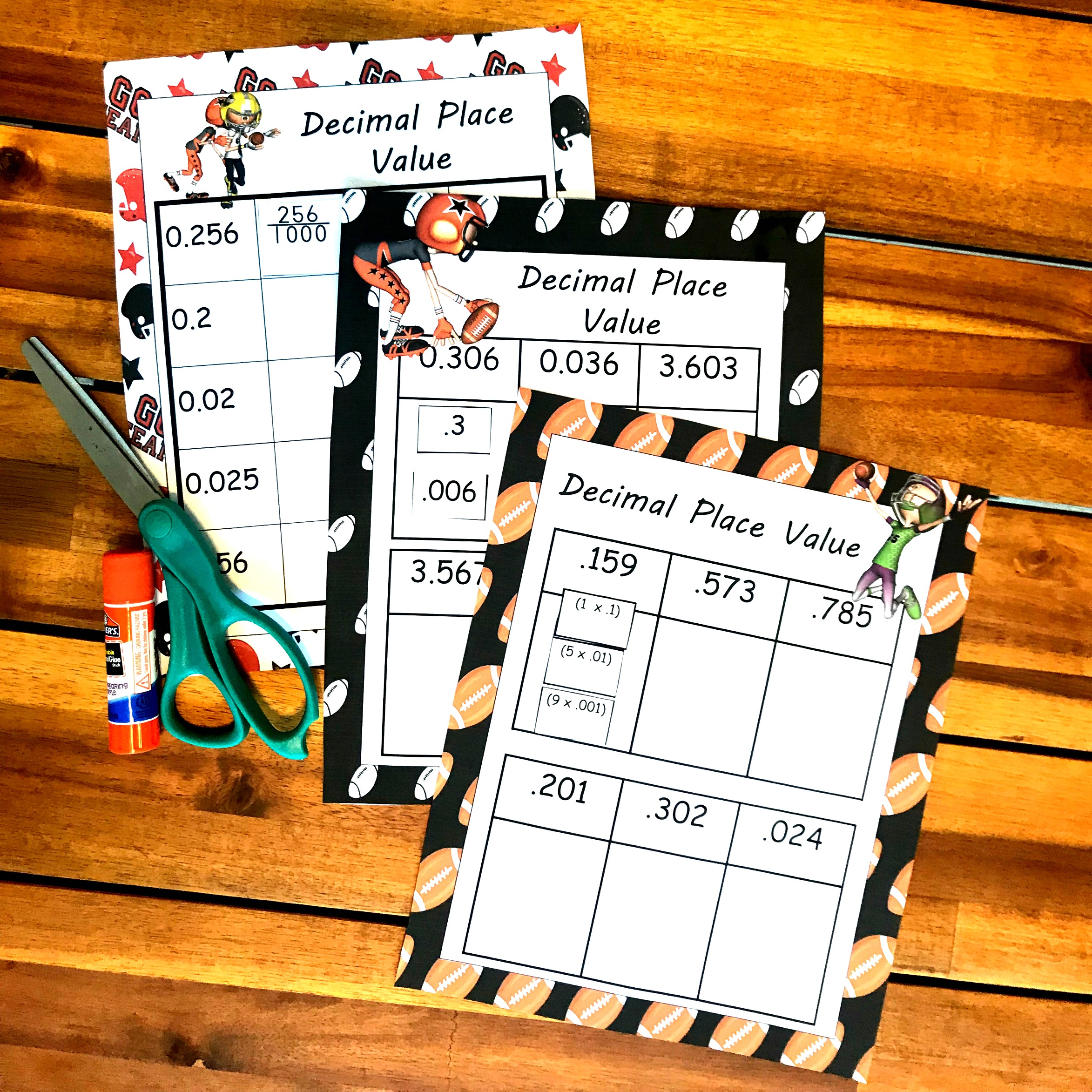# Free Cut and Paste Decimal Place Value Worksheets (Expanded Form)

Your kids will enjoy these Decimal Place Value Worksheets with a football theme. They work on expanded form and changing decimals to fractions!

It’s crazy. The footballs have been sitting in baskets all summer. But now that September has come and football is on ALL the time, the dust has been dusted off those balls.

Now football games are happening in the backyard, out front on the street with Daddy, and they practice tackling on the trampoline.

So with the football craze going on, I knew I needed some football-themed math activities to spice up my oldest’s math time.

So here are some decimal place value worksheets with a football theme.

## Prep-work For Decimal Place Value Worksheets

There is no prep work required with these worksheets.

Just print off the sheets you want to do, and then grab some glue and scissors! Now you are ready for some decimal place value work!

Decimal Place Value Worksheets

There are three different worksheets that focus on different skills.

### Decimal Expanded Form

The first worksheet focuses on expanded form. The students are given the number 0.306 and are asked to show it in expanded form.

If you haven’t worked on what each place of a decimal represents, you will need to start here.

Activities like modeling decimals or  Decimal of the DAY   will help children visualize and develop an understanding of decimals!

But if your children have already explored and have developed a better understanding of decimals, it is time to look at each digit and figure out what they stand for.

The first digit that isn’t a zero is the three. It is in the tenths place so we look for a number that shows three- tenths. (.3)

The next number we come to is a six. It is in the thousandths place, so we find the number that shows the six in the thousandths place. (.006)

Now we glue .3 and .006 underneath 0.306 and we have shown the expanded form.

### Expanded Form with a Twist

Before you have your children work through these sheets, I would highly suggest working on multiplying decimals by whole numbers.

Once they have explored this concept, you can move to this cut and paste decimal expanded form sheet.

For example, 0.159 can be written like this…..

1 x .1 (the one is in the tenths place)

5 x .01 (the five is in the hundredths place)

9 x .001 (the nine is in the thousandths place)

## Decimal to Fractions:

The last sheet is an easy one. It is simply changing decimals to fractions.

In this example, the children are asked to change two hundred, fifty-six thousandths to a decimal. Since our decimal is in the thousandths place, the denominator will be 1,000 and the numerator is 256.

These simple decimal place value worksheets are a great way to work on decimals.

You’ve Got This,

Rachel

You may also like:

The Ultimate Decimal Bundle